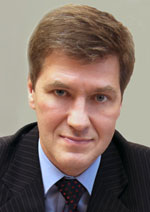Publications
Editor-in-ChiefNikiforov
D.Sc., Prof.
Partners

doi: 10.17586/2226-1494-2015-15-6-1147-1154

METHOD OF TRAINING EXAMPLES IN SOLVING INVERSE ILL-POSED PROBLEMS OF SPECTROSCOPY

V. S. Sizikov, A. V. Stepanov

Read the full article';
Article in English

For citation: Sizikov V.S., Stepanov A.V. Method of training examples in solving inverse ill-posed problems of spectroscopy. Scientific and Technical Journal of Information Technologies, Mechanics and Optics, 2015, vol. 15, no. 6, pp. 1147–1154.

Abstract

Subject of Study.The paper deals with further development of the method of computational experiments for solving ill-posed problems, e.g., the inverse spectroscopy problem. This method produces an effective (nonoverstated) estimate for solution error of the first-kind equation. Method of Research.  An equation is solved by the Tikhonov regularization method. We have obtained nonoverstated estimate for solution error and a new principle for choosing the regularization parameter on the basis of the truncating singular number spectrum of an operator. It is proposed to estimate the truncation magnitude by results of solving model (training, learning) examples close to an initial example (problem). This method takes into account an additional information about the solution. Main Results. We have derived a new, more accurate estimate for regularized solution error using the truncation parameter g. Ways for determining g according to the results of solving model examples are proposed. The method of modeling or training is applied to solving the inverse spectroscopy problem (restoration of a fine spectrum structure by solving integral equation on the basis of an experimental spectrum and the spread function of a spectral device). The method makes it possible to resolve close lines and select weak lines. Practical Relevance. The proposed method can be used to restore smoothed and noisy spectra, in other words, to enhance the resolution of spectral devices by mathematical and computer processing of experimental spectra.

Keywords: ill-posed problems, Tikhonov regularization, solution error, method of training examples, inverse problem of spectroscopy, integral equation, spread function of spectral device, measured spectrum, training spectra, restored spectrum.

Acknowledgements. This work was supported by the Russian Foundation for Basic Research (RFBR), grant №13-08-00442.

References

1. Sizikov V.S. Application of modelling for solution of ill-posed problems. Electronic Modeling, 1981, no. 6, pp. 3–8 (in Russian).
2. Sizikov V.S. Generalized method for measurement data reduction. I. Processing of tonal (narrow-band) signals. Electronic Modeling, 1992, vol. 13, no. 4, pp. 618–633.
3. Sizikov V.S. Generalized measurement reduction method. III. Results of numerical simulation. Electronic Modeling, 1993, vol. 13, no. 6, pp. 1023–1035.
4. Verlan' A.F., Sizikov V.S. Integral Equations: Methods, Algorithms, Programs. Kiev, Naukova Dumka, 1986, 544 p. (in Russian).
5. Sizikov V.S. Inverse Applied Problems and MatLab. St. Petersburg, Lan' Publ., 2011, 256 p. (in Russian).
6. Sizikov V.S. Integral Equations and MatLab in Tomography, Iconics, and Spectroscopy Problems. St. Petersburg-Saarbrücken, LAP, 2011, 252 p. (in Russian).
7. Verlan' A.F., Sizikov V.S., Mosentsova L.V. Method of computation experiments for solving integral equations in the inverse spectroscopy problem. Electronic Modeling, 2011, vol. 33, no. 2, pp. 3–12 (in Russian).
8. Sizikov V.S., Krivykh A.V. Reconstruction of continuous spectra by the regularization method using model spectra. Optics and Spectroscopy, 2014, vol. 117, no. 6, pp. 1010–1017. doi: 10.1134/S0030400X14110162
9. Tikhonov A.N., Goncharsky A.V., Stepanov V.V., Yagola, A.G. Numerical Methods for the Solution of
Ill-Posed Problems. Dordrecht, Kluwer, 1995, 232 p.
10. Voskoboinikov Yu.E., Preobrazhenskii N.G., Sedel’nikov A.I. Mathematical Processing of Experiment in Molecular Gas Dynamics. Novosibirsk, Nauka, 1984, 240 p. (in Russian).
11. Engl H.W., Hanke M., Neubauer A. Regularization of Inverse Problems. Dordrecht, Kluwer, 1996, 328 p.
12. Petrov Yu.P., Sizikov V.S. Well-Posed, Ill-posed, and Intermediate Problems with Applications. Leiden-Boston, VSP, 2005, 234 p.
13. Leonov A.S., Yagola A.G. Adaptive optimal algorithms for ill-posed problems with sourcewise represented solutions. Computational Mathematics and Mathematical Physics, 2001, vol. 41, no. 6, pp. 807–824.
14. Bakushinsky A., Goncharsky A. Ill-Posed Problems: Theory and Applications. Dordrecht, Kluwer, 1994, 268 p.
15. Kojdecki M.A. New criterion of regularization parameter choice in Tikhonov's method. Biuletyn WAT (Biul. Mil. Univ. Technol.), 2000, vol. 49, no. 1(569), pp. 47–126.
16. Sizikov V.S. On discrepancy principles in solving ill-posed problems. Computational Mathematics and Mathematical Physics, 2003, vol. 43, no. 9, pp. 1241–1259.
17. Sizikov V.S. Further development of the new version of a posteriori choosing regularization parameter in  ill-posed problems. International Journal of Artificial Intelligence, 2015, vol. 13, no. 1, pp. 184–199.
18. Morozov V.A. Methods for Solving Incorrectly Posed Problems. NY, Springer, 1984, 240 p.
19. Starkov V.N. Constructive Methods of Computational Physics in Interpretation Problems. Kiev, Naukova Dumka, 2002, 264 p. (in Russian).
20. Gfrerer H. An a posteriori parameter choice for ordinary and iterated Tikhonov regularization of ill-posed problems leading to optimal convergence rates. Mathematics of Computation, 1987, vol. 49, no. 180, pp. 507–522.
21. Golub G.H., Heath M., Wahba G. Generalized cross-validation as a method for choosing a good ridge parameter. Technometrics, 1979, vol. 21, no. 2, pp. 215–222.
22. Hansen P.C., O'Leary D.P. The use of the L-curve in the regularization of discrete ill-posed problems. SIAM Journal of Scientific Computing, 1993, vol. 14, no. 6, pp. 1487–1503.
23. Voskoboinikov Yu.E., Mukhina I.N. Local regularizing algorithm for high-contrast image and signal restoration. Optoelectronics, Instrumentation and Data Processing, 2000, no. 3, pp. 41–48.